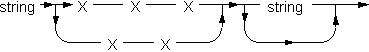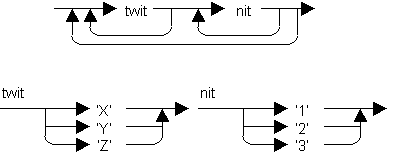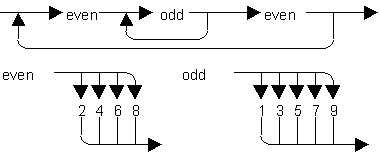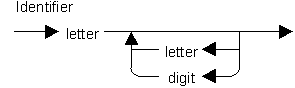Exercises
Syntax Diagrams

1. Consider the syntax diagram at right. Which of the following is not a valid string according to this diagram?
 A. X B. XX C. XXXX D. XXXXX E. XXXXXXX2. Given the syntax diagrams at right, tell which the following are valid "dwits."
 a. XYZ b. 123 c. X1 d. 23Y e. XY12 f. Y2Y g. ZY2 h. XY23X13. Given the syntax diagrams at right, mark the following as valid or invalid.
 a. 212 b. 333 c. 0330 e. 61357984. Mark the following as valid or invalid identifiers
 a. Item#1 b. data c. Y d. ISet e. investment f. Bin-2 g. nums h. Sq.Ft.Continue to:  Unit 3 / Prev / Next